SLVSD74D December   2015  – December 2019

PRODUCTION DATA.

1. Features
2. Applications
3. Description
1.     Device Images
4. Revision History
5. Device Comparison Table
6. Pin Configuration and Functions
7. Specifications
8. Detailed Description
1. 8.1 Overview
2. 8.2 Functional Block Diagram
3. 8.3 Feature Description
1. 8.3.1 Pin Current and Voltage Conventions
2. 8.3.2 Accurate Current Sense
3. 8.3.3 Adjustable Current Limit
4. 8.3.4 Inductive-Load Switching-Off Clamp
5. 8.3.5 Fault Detection and Reporting
6. 8.3.6 Full Diagnostics
1. 8.3.6.1 Short-to-GND and Overload Detection
2. 8.3.6.2 Open-Load Detection
3. 8.3.6.3 Short-to-Battery Detection
4. 8.3.6.4 Reverse Polarity Detection
5. 8.3.6.5 Thermal Fault Detection
7. 8.3.7 Full Protections
4. 8.4 Device Functional Modes
9. Application and Implementation
1. 9.1 Application Information
2. 9.2 Typical Application
10. 10Power Supply Recommendations
11. 11Layout
1. 11.1 Layout Guidelines
2. 11.2 Layout Examples
12. 12Device and Documentation Support
13. 13Mechanical, Packaging, and Orderable Information

• PWP|16
• PWP|16

#### 9.2.3 Application Curves

Figure 39 shows a test example of soft-start when driving a big capacitive load. Figure 40 shows an expanded waveform of the output current.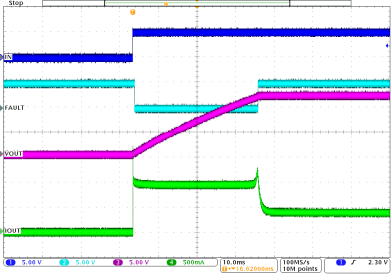VS = 12 V INx = ↑ Current limit = 1 A Load current = 0.4 A CL = 2.3 mF CH1 = INx CH2 = FAULT CH3 = output voltage CH4 = output current
Figure 39. Driving a Capacitive Load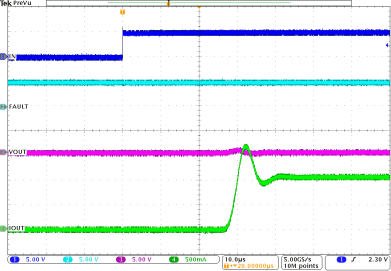VVS = 12 V INx = ↑ Current limit = 1 A Load current = 0.4 A CL = 2.3 mF CH1 = INx CH2 = FAULT CH3 = output voltage CH4 = output current
Figure 40. Driving a Capacitive Load, Expanded Waveform

Figure 41 shows a test example of PWM-mode driving. Figure 42 shows the expanded waveform of the rising edge. Figure 43 shows the expanded waveform of the falling edge.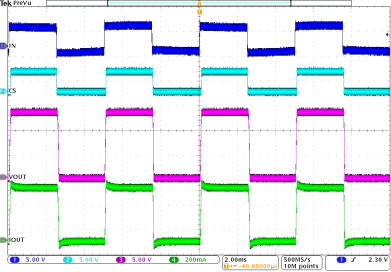VVS = 13.5 V INx = 200-Hz PWM at 50% duty cycle CH1 = INx signal CH2 = CS voltage CH3 = output voltage CH4 = output current
Figure 41. PWM Signal Driving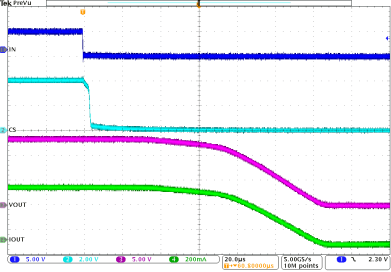VVS = 13.5 V INx = 200-Hz PWM at 50% duty cycle CH1 = INx signal CH2 = CS voltage CH3 = output voltage CH4 = output current
Figure 43. Expanded Waveform of Falling Edge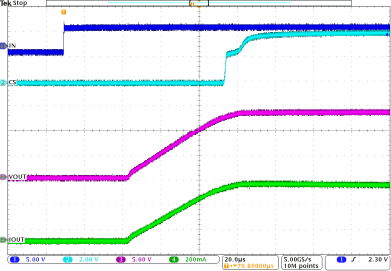VVS = 13.5 V INx = 200-Hz PWM at 50% duty cycle CH1 = INx signal CH2 = CS voltage CH3 = output voltage CH4 = output current
Figure 42. Expanded Waveform of Rising Edge